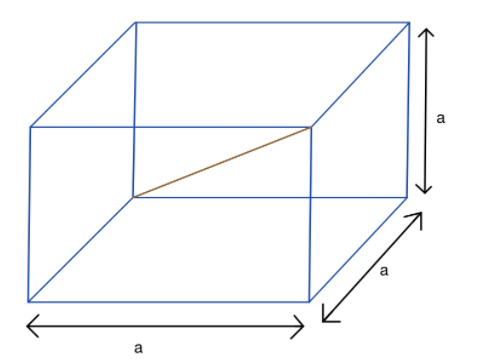QuestionAnswers

# The volume of a cube (in cubic cm) plus three times the total length of its edges ( in cms) is equal to twice its area (in sq. cm). The length of its diagonal is(A). 6(B). $6\sqrt{3}$(C). $3\sqrt{6}$(D). $6\sqrt{6}$Hint: In this question it is given that the volume of a cube (in cubic cm) plus three times the total length of its edges ( in cms) is equal to twice its area (in sq. cm). Then we have to find the length of its diagonal. So to understand it in better ways we have to draw the diagram,So to find the solution, first we have to find the volume and the surface area of the given cube and after that by the given condition we are able to find the length diagonal.
Where, we have to know some formulas,
Volume of a cube(V)=$\left( \text{side} \right)^{3}$,......(1)
The total surface area(A)=$6\times \left( \text{side} \right)^{2}$.......(2)
And the diagonal of a cube =$\sqrt{3} \times \text{side}$.........(3)

Complete step-by-step solution:
Let the side of the cube be a cm,
Now since the cube has 6 faces and each face is a square of side a cm.
Therefore, for the 6 faces area will be, $A=6a^{2}\ cm^{2}$,
Then the total surface area of a cube $(A)=a^{2}\ cm^{2}$........(1)
And the volume$(V)=a^{3}\ cm^{2}$........(2)
Now as we can see a cube has 12 edges and each of length a.
Then we can say that the total length of its edges(S)=12a cm…..(3)

In the question it is given, the volume of a cube (in cubic cm) plus three times the total length of its edges ( in cms) is equal to twice its area (in sq. cm),
i,e $V+3S=2A$..........(4)
Now by putting the value of V, S an A in equation (4), we get,
$a^{3}+3\left( 12a\right) =2\left( 6a^{2}\right)$
$\Rightarrow a^{3}+36a=12a^{2}$
$\Rightarrow a^{3}-12a^{2}+36a=0$
$\Rightarrow a(a^{2}-12a+36)=0$
$\Rightarrow a(a^{2}-2\cdot a\cdot 6+6^{2})=0$
Now as we know that $\left( x-y\right)^{2} =x^{2}-2xy+y^{2}$,
So by using the identity, we can write the above equation as,
$a\left( a-6\right)^{2} =0$
$a\left( a-6\right) \left( a-6\right) =0$
Therefore, either, a=0, which is not possible.
Or, $a-6=0\Rightarrow a=6$
Therefore, the diagonal of a cube= $\sqrt{3} a$
=$\sqrt{3} \times 6$ cm
=$6\sqrt{3}$ cm
Hence the correct option is option B.

Note: You might be thinking how can we get the expression for surface area, so let's find it.
As we know that a cube has 6 surfaces and each surface is in the shape of a square, where the length of each edge is a. Then you can easily say that the area of this surface is $a^{2}$, so from here we can easily find the total surface area is 6 times of the area of one surface, i.e, $a^{2}$. Also you have to know that, all the diagonals of a cube are equal.

View Notes
CBSE Class 8 Maths Chapter 7 - Cubes and Cube Roots FormulasVolume of a Triangular Prism FormulaCube Root of 9261Cube Root of 216Cube Root of 64Cube Root of 2Cube Root of 4Cube Root of 343Cube Root of 512Cube Root of 2197Important Questions for CBSE Class 6 English A Pact with The Sun Chapter 8 - A Pact with the SunCBSE Class 8 Science Reaching The Age of Adolescence WorksheetsImportant Questions for CBSE Class 8 Maths Chapter 7 - Cubes and Cube RootsImportant Questions for CBSE Class 7 Social Science - Social and Political Life Chapter 8 - A Shirt In The MarketImportant Questions for CBSE Class 6 English A Pact with The Sun Chapter 1 - A Tale of Two BirdsImportant Questions for CBSE Class 6 English Honeysuckle Chapter 8 - A Game of ChanceImportant Questions for CBSE Class 8 Social Science Our Past 3 Chapter 4 - Tribals, Dikus and the Vision of a Golden AgeImportant Questions for CBSE Class 6 English A Pact with The Sun Chapter 2 - The Friendly MongooseImportant Questions for CBSE Class 8 English Honeydew Chapter 3 - Glimpses of the PastImportant Questions for CBSE Class 6 English A Pact with The Sun Chapter 6 - The Monkey and the CrocodileCBSE Class 10 Hindi A Question Paper 2020Hindi A Class 10 CBSE Question Paper 2009Hindi A Class 10 CBSE Question Paper 2015Hindi A Class 10 CBSE Question Paper 2016Hindi A Class 10 CBSE Question Paper 2012Hindi A Class 10 CBSE Question Paper 2010Hindi A Class 10 CBSE Question Paper 2007Hindi A Class 10 CBSE Question Paper 2013Hindi A Class 10 CBSE Question Paper 2008Hindi A Class 10 CBSE Question Paper 2014RD Sharma Solutions for Class 9 Maths Chapter 18 - Surface Area and Volume of a Cuboid and CubeNCERT Solutions for Class 8 Maths Chapter 7 Cubes and Cube RootsRD Sharma Solutions for Class 9 Maths Chapter 21 - Surface Area and Volume of a SphereRD Sharma Solutions for Class 9 Maths Chapter 19 - Surface Area and Volume of A Right Circular CylinderRS Aggarwal Class 8 Mathematics Solutions for Chapter-18 Area of a Trapezium and a PolygonRD Sharma Class 8 Solutions Chapter 4 - Cube and Cube Roots (Ex 4.2) Exercise 4.2NCERT Solutions for Class 8 Maths Chapter 7 Cubes and Cube Roots in HindiRS Aggarwal Class 8 Mathematics Solutions for Chapter-20 Volume and Surface Area of SolidsNCERT Exemplar for Class 8 Maths Solutions Chapter 3 Square-Square Root & Cube-Cube RootRD Sharma Solutions for Class 9 Maths Chapter 20 - Surface Area and Volume of A Right Circular Cone